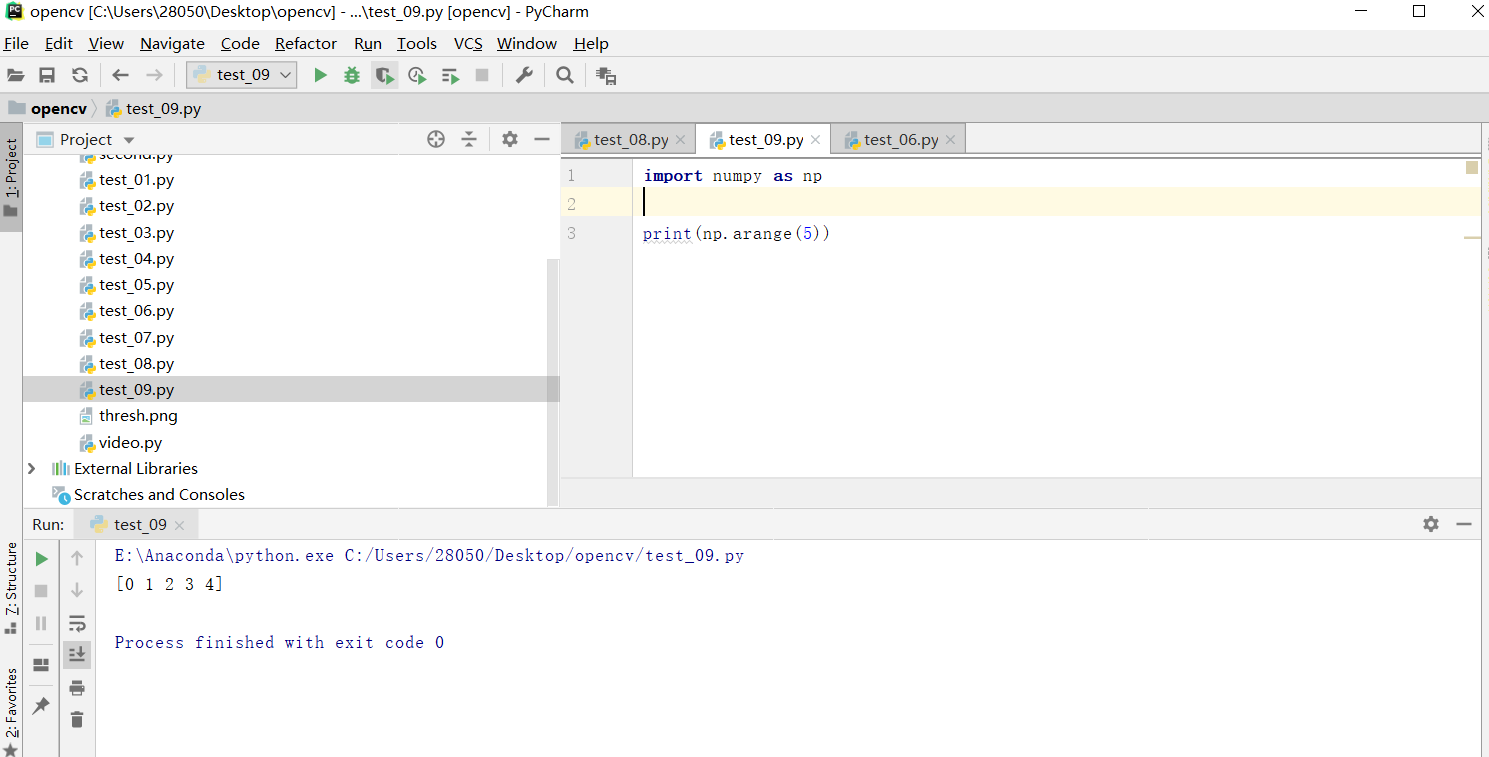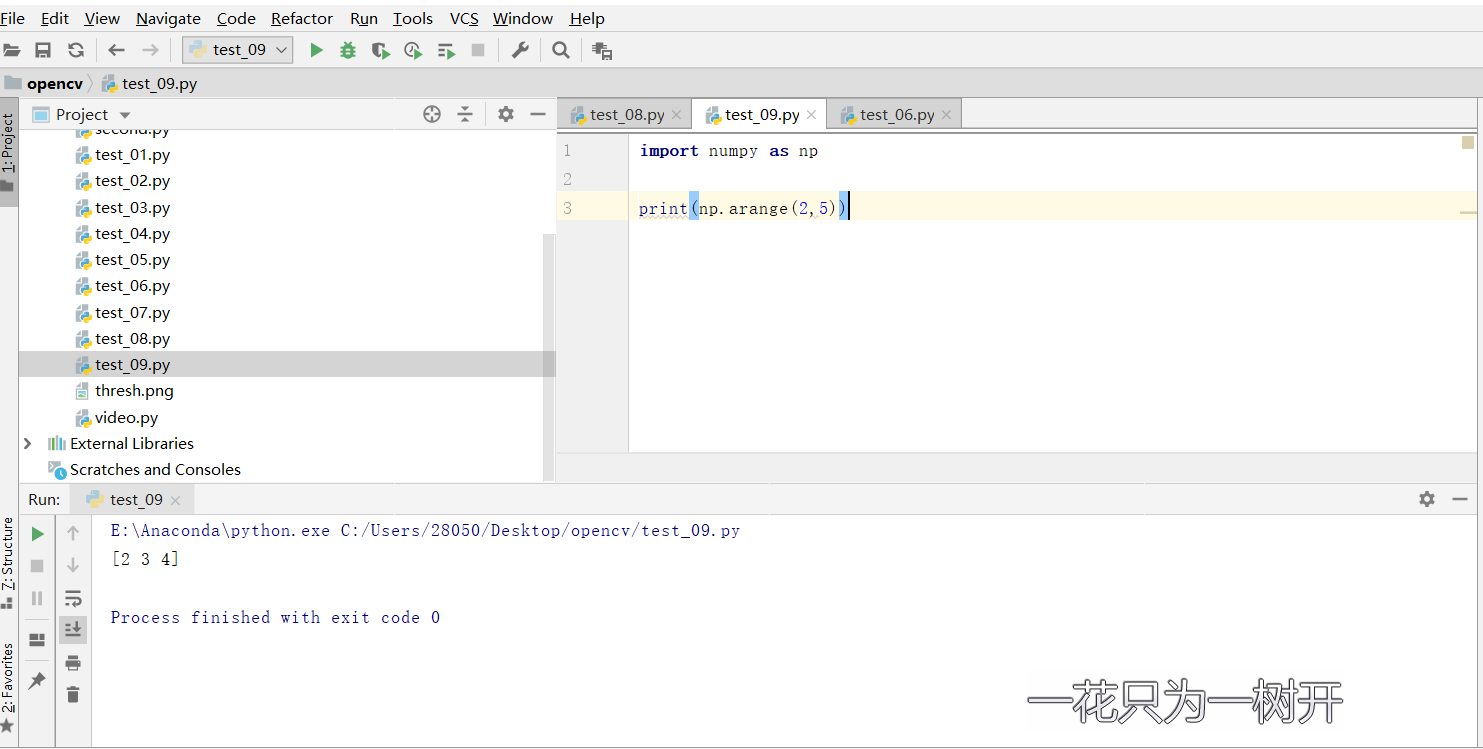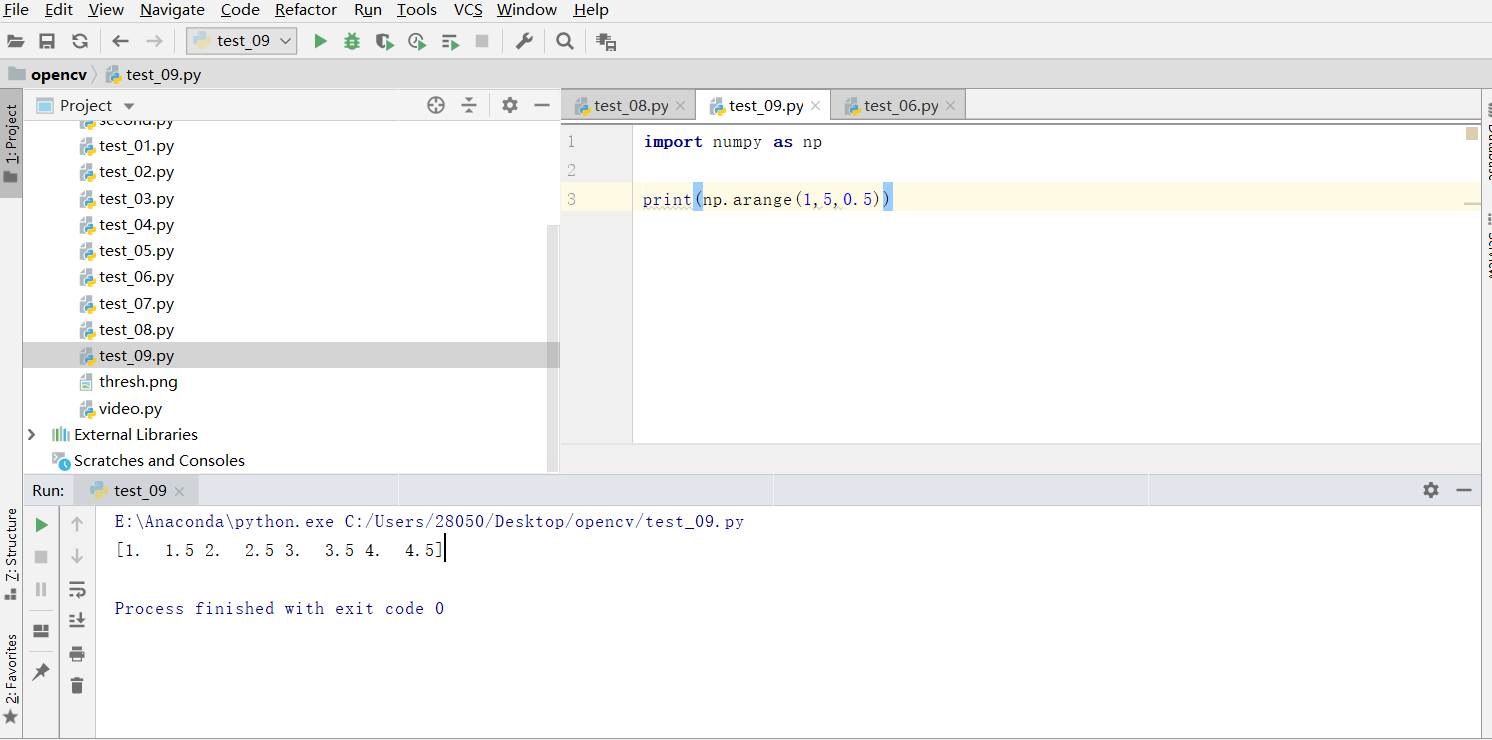• numpy.arrange
千次阅读
2021-12-11 17:13:18

numpyarrange用于生成等差数组。
代码示例1

import numpy as np

# 等差数组的区间是[0, 5)，默认步长为1
a = np.arange(0, 5)
print(a)


执行结果：

[0 1 2 3 4]


代码示例2

import numpy as np

# 等差数组的区间是[0, 50)，步长为10
a = np.arange(0, 50, 10)
print(a)


执行结果：

[ 0 10 20 30 40]python
更多相关内容
• ## numpy.arrange函数知识大全

万次阅读 多人点赞 2019-04-15 21:11:04
numpy.arrange函数知识大全numpy.arrange函数作用 numpy.arrange函数作用 numpy.arrange函数的作用是生成带起点和终点的特定步长的排列。 根据函数的参数的个数分为以下几种情况: 1.只有一个参数，则这个参数为...

# numpy.arrange函数作用

numpy.arrange函数的作用是生成带起点和终点的特定步长的排列。
根据函数的参数的个数分为以下几种情况:
1.只有一个参数，则这个参数为终点。起点默认为0,步长默认为1.
numpy.arange(5)的结果如图:2.有两个参数，第一个参数为起点，第二个参数为终点，步长默认为1。默认取值左闭右开。
numpy.arange(2,5)结果如图：3.有三个参数，第一个参数为起点，第二个参数为终点，第三个参数为步长。步长支持小数。numpy.arange(1,5,0.5)结果如图：展开全文• 函数：arrange() 函数说明：arange([start,] stop[, step,], dtype=None) 根据 start 与 stop 指定的范围以及 step 设定的步长，生成一个 ndarray。 返回array 类型对象。 >>> range() 中的步长不能为小数，但是...函数：range()

• 函数说明： range(start, stop[, step]) -> range object，根据 start 与 stop 指定的范围以及 step 设定的步长，生成一个序列。
• 参数含义：
1. start: 计数从 start 开始。默认是从 0 开始。例如 range（5）等价于 range（0， 5）;
2. end: 开始到 end 结束，不包括 end. 例如：range（0， 5） 是 [0, 1, 2, 3, 4] 没有 5
3. scan：每次跳跃的间距，默认为 1。例如：range（0， 5） 等价于 range(0, 5, 1)
4. range 多用作循环，range（0,10）返回一个 range 对象，如想返回一个 list，前面加上 list 转换

函数返回的是一个 range object

>>> 

range中的setp 不能使float,所有range不能生成小数。

函数：arrange()

• 函数说明：arange([start,] stop[, step,], dtype=None) 根据 start 与 stop 指定的范围以及 step 设定的步长，生成一个 ndarray。 返回array 类型对象。
>>> 
• range() 中的步长不能为小数，但是numpy.arange() 中的步长可以为小数
>>> 
展开全文numpy whl
• array：创建数组dtype：指定数据类型empty：创建数据接近0zeros：创建数据全为0ones：创建数据全为1arrange：按指定范围创建数据linspace：创建线段 import numpy as np #为了方便使用numpy 采用np简写 array = ...

参考链接： Python中的numpy.empty

array：创建数组dtype：指定数据类型empty：创建数据接近0zeros：创建数据全为0ones：创建数据全为1arrange：按指定范围创建数据linspace：创建线段

import numpy as np #为了方便使用numpy 采用np简写

array = np.array([[1,2,3],[2,3,4]])  #列表转化为矩阵

print(array)

"""

[[1 2 3]

[2 3 4]]

"""

print('number of dim:',array.ndim)  # 维度

# number of dim: 2

print('shape :',array.shape)    # 行数和列数

# shape : (2, 3)

print('size:',array.size)   # 元素个数

# size: 6

a = np.empty((3,4)) # 数据为empty，3行4列,创建全空数组, 其实每个值都是接近于零的数

"""

array([[  0.00000000e+000,   4.94065646e-324,   9.88131292e-324,

1.48219694e-323],

[  1.97626258e-323,   2.47032823e-323,   2.96439388e-323,

3.45845952e-323],

[  3.95252517e-323,   4.44659081e-323,   4.94065646e-323,

5.43472210e-323]])

"""

a = np.zeros((3,4)) # 数据全为0，3行4列

"""

array([[ 0.,  0.,  0.,  0.],

[ 0.,  0.,  0.,  0.],

[ 0.,  0.,  0.,  0.]])

"""

a = np.ones((3,4),dtype = np.int)   # 数据为1，3行4列

"""

array([[1, 1, 1, 1],

[1, 1, 1, 1],

[1, 1, 1, 1]])

"""

注意arange和linspace的区别

a = np.arange(1,106,5.5).reshape((5,4))  # 区间为[1, 106) 的数据，步长为5.5

>>> a

array([[  1. ,   6.5,  12. ,  17.5],

[ 23. ,  28.5,  34. ,  39.5],

[ 45. ,  50.5,  56. ,  61.5],

[ 67. ,  72.5,  78. ,  83.5],

[ 89. ,  94.5, 100. , 105.5]])

a = np.linspace(1,105.5,20).reshape((5,4))  # 开始端1，结束端105.5，且分割成20个数据，生成线段

>>> a

array([[  1. ,   6.5,  12. ,  17.5],

[ 23. ,  28.5,  34. ,  39.5],

[ 45. ,  50.5,  56. ,  61.5],

[ 67. ,  72.5,  78. ,  83.5],

[ 89. ,  94.5, 100. , 105.5]])

展开全文• arrange函数用于创建等差数组。 返回一个有起点和终点固定长的list e.g.[1, 2, 3],起点是1，终点是5，步长是1。步长相当于等差数列中的公差。 参数：可以接受1、2、3个参数。 注意：如果起始值大于终点值，会...
• 转载：https://blog.csdn.net/yuanxiang01/article/details/78702123python
• -1, -2, -3, -4, -5, -6, -7, -8, -9] >>> range(0) [] >>> range(1, 0) [] numpy.arange([start, ]stop, [step, ]dtype=None) 在给定的时间间隔内返回均匀间隔的值。 在半开区间[start， stop）内产生值 ...python
• 关于这个numpy函数每次用，每次都要查资料，所以就记下笔记，在用就来查自己的笔记~~ 一、NumPy(Numerical Python) 是 Python 语言的一个扩展程序库，支持大量的维度数组与矩阵运算，此外也针对数组运算提供大量的...
• 1、numpy中的数组 （1）数组维数 import numpy as np arr1d = np.arange(24).reshape(24) print('一维数组：', arr1d) arr2d = np.arange(24).reshape((4, 6)) print('二维数组：\n', arr2d) arr3d = np.a...python transpose
• class 'numpy.ndarray'> 故将numpy的ndarray类型转为int (或者你需要的类型，此处为int） 使用 list(m).tolist() 的方法得到如下的结果 63 <class 'int'> Ok，问题成功解决。 ...python
• python 中 np.arange()的使用 - 博二爷 - 博客园python
• array：创建数组 dtype：指定数据类型 empty：创建数据接近0 zeros：创建数据全为0 ...import numpy as np #为了方便使用numpy 采用np简写 array = np.array([[1,2,3],[2,3,4]]) #列表转化为矩阵 print(arra...
• 转载：https://www.cnblogs.com/ghllfl/p/8487692.htmlnp.arange()经常用，其用法总结如下：np.arange(0,60,2)生成从0到60的步距为2的数组，其中0为初始值，60为终值，2步...import numpy as npprint(np.arange(0,6...
• numpy reshape方法 import numpy as np array = np.arange(8) print("Original array : \n", array) # shape array with 2 rows and 4 columns array = np.arange(8).reshape(2, 4) print("\narray reshaped ...
• import numpy as np &gt;&gt;&gt; t1 = np.arange(0, 0.01*57, 0.01) &gt;&gt;&gt; t1.shape (58L,) 但是如果用同样的方法，可以得到正确的长度为58的、从0开始的、...python 浮点数精度 decimal
• 参考： numpy常用函数之arange函数 np.arange函数 Python 基础——range() 与 np.arange()
• ## np.arrange用法

千次阅读 2020-12-11 07:14:37
np.arange()经常用，其用法总结如下：np.arange(0,60,2)生成从0到60的步距为2的数组，其中0为初始值，60为终值，2步距，np.arange(60)生成从0到59的默认步距为1的数组Python程序示例：import numpy as npprint(np.a...
• 本文实例讲述了python+numpy实现的基本矩阵操作。分享给大家供大家参考，具体如下：#! usr/bin/env python# coding: utf-8# 学习numpy中矩阵的代码笔记# 2018年05月29日15:43:40# 参考网站：...
• 这时，我们经常用到 Numpy 中的 linspace 和 arange 函数。然而稍不注意，两者就容易用错，特别是 linspace，现在对两者的用法和区别说明如下，并指出两者容易用错的地方。 1、arange 函数的用法 为了便于分析，我们...linspace python
• 主要介绍了浅谈Python中range与Numpy中arange的比较，具有很好的参考价值，希望对大家有所帮助。一起跟随小编过来看看吧
• 3维变为2维 a = np.arange(24).reshape(2,3,4) array([[[ 0, 1, 2, 3], [ 4, 5, 6, 7], [ 8, 9, 10, 11]], [[12, 13, 14, 15], [16, 17, 18, 19], [20, 21, 22, 23]]]) a.reshape(2*3,4) ...
• 一、Numpy是什么Numpy代表"Numerical Python’，那么Numpy是什么呢？Numpy是一个python的数字库NumPy包含多维数组和矩阵数据结构数组结构支持与三角、统计、和线性代数相关的大部分函数。Numeric和Nuarray的扩展...
• k in iteract) # Re-arrange ypred and ytrue: ypred, ytrue = zip(*ya) ypred = [np.concatenate(tuple(k)) for k in np.split(np.array(ypred), nfeat)] ytrue = [np.concatenate(tuple(k)) for k in np.split(np...
• concatenate()函数是NumPy包中的函数。此函数实质上将NumPy数组组合在一起。此功能基本上用于沿指定轴连接两个或多个相同形状的数组。需要牢记以下几点：NumPy的concatenate()不同于传统的数据库联接。就像堆叠NumPy...
• numpy简介 Numpy 是一个专门用于矩阵化运算、科学计算的开源Python库，Numpy将Python相当于变成一种免费的更强大的Matlab系统 优势： 1、强大的 ndarray 多维数组结构 2、成熟的函数库 3、用于整合C/C++和Fortran...
• ## 3.3 numpy

千次阅读 2021-07-20 07:15:26
import numpy as np '{}'.format(np.typeDict.values()) "dict_values([<class 'numpy.bool_'>, <class 'numpy.bool_'>, <class 'numpy.int8'>, <class 'numpy.int8'>, <class ...python 大数据 java
• numpy.reshape()函数在NumPy包中可用。顾名思义, 整形意味着”形状变化”。 numpy.reshape()函数可帮助我们在不更改数据的情况下获得数组的新形状。有时, 我们需要将数据从宽到长整形。因此, 在这种情况下, 我们必须......